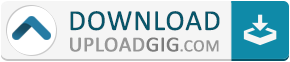#Do not remember me!

### Categories» » » Numerical Python in Astronomy and Astrophysics: A Practical Guide to Astrophysical Problem Solving

## Numerical Python in Astronomy and Astrophysics: A Practical Guide to Astrophysical Problem SolvingEnglish | 2021 | ISBN: 3030703460 | 257 pages | pdf, epub | 88.08 MB

This book provides a solid foundation in the Python programming language, numerical methods, and data analysis, all embedded within the context of astronomy and astrophysics. It not only enables students to learn programming with the aid of examples from these fields but also provides ample motivation for engagement in independent research. The book opens by outlining the importance of computational methods and programming algorithms in contemporary astronomical and astrophysical research, showing why programming in Python is a good choice for beginners. The performance of basic calculations with Python is then explained with reference to, for example, Kepler’s laws of planetary motion and gravitational and tidal forces. Here, essential background knowledge is provided as necessary. Subsequent chapters are designed to teach the reader to define and use important functions in Python and to utilize numerical methods to solve differential equations and landmark dynamical problems in astrophysics. Finally, the analysis of astronomical data is discussed, with various hands-on examples as well as guidance on astronomical image analysis and applications of artificial neural networks.Name:* E-Mail: Security Code: *